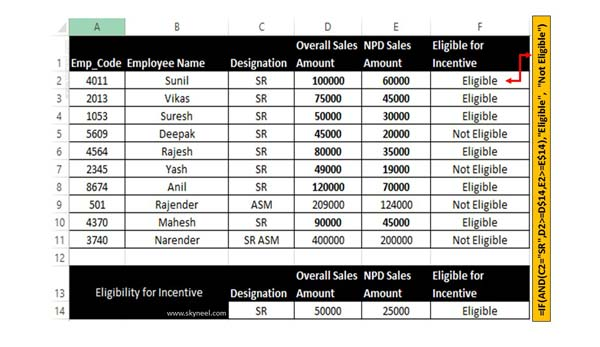# How to use Excel Nested AND Function to check multiple criteria

Excel Nested AND Function is a logical function which allows you to check multiple criteria and generate result which match all condition.

The Nested AND Function Excel in a logical function which play an important role to check multiple conditions and provide the result. IF, OR, NOT function also are important logical functions which allows us to match the complex criteria and provide the result which match the condition.

IF Function is widely used around the world and too much popular logical function. This function returns first value if given first criteria is evaluates to TRUE otherwise evaluates to FALSE. AND Function allows you to return TRUE if all the given criteria are TRUE otherwise return FALSE if one or more argument is FALSE. If you have multiple criteria then you can use IF Statement with AND, OR function as per your need. AND, OR Function along with IF function allows you to resolve complex criteria and provide the result which match the condition. So, let start discuss how to use IF Statement with AND function in Excel.

### IF Function in Excel

It is one of the most useful logical function of MS Excel. This function is used to check given condition is true or false. If the given condition is true, then function will return one value, if the condition is false, the function will do something else.

Syntax of IF function

IF (logic test, value if true, value if false)

The logic test compare between given two values by using any comparison operator. The body part of this function are also known as arguments.

### AND Function in Excel

Returns TRUE, if all given values or arguments are TRUE. Returns FALSE if any single or multiple arguments are FALSE.

Syntax of AND function

AND (logical1,logical2, …)

Logical1, logical2, logical3, … are 1 to 255 conditions.

If you are using AND with if function then if both condition are true then result will be true, if any one or both condition result is false then the result will be false.

For eg: If you have a database in which you want to know who is eligible for incentive. You have three criteria who match these criteria is eligible for incentive.

1. Designation = SR
2. Monthly Overall Sales Amount is greater than or equal to Rs. 50000
3. Monthly New Product (NPD) Sales Amount is greater than or equal to Rs. 25000

## Steps to use Excel Nested AND Function to check multiple criteria

If you have multiple criteria and you have to fulfil all the condition in that situation you must have to use Nested logical AND with IF function in Excel.Step 1: Create the following given database in your active worksheet.

Step 2: To know who is eligible for incentive you must have to use IF Function with AND function in F Column. You have to type given function if F2.

=IF(AND(C2=”SR”,D2>=D\$14,E2>=E\$14),”Eligible”, “Not Eligible”)

After typing the formula press enter to get the result. Now drag the given function from F2:F11 with the help of Fill Handle button.